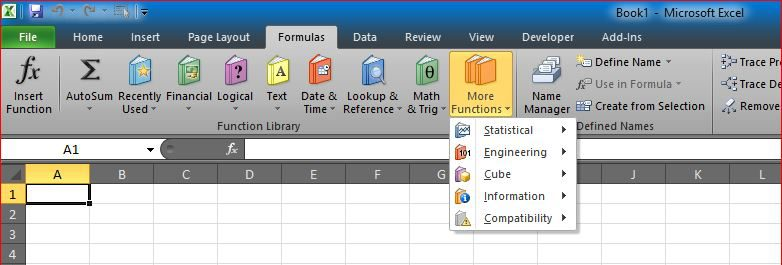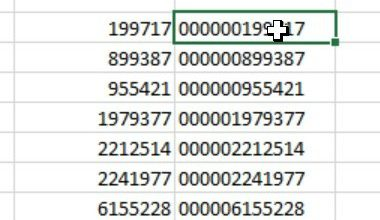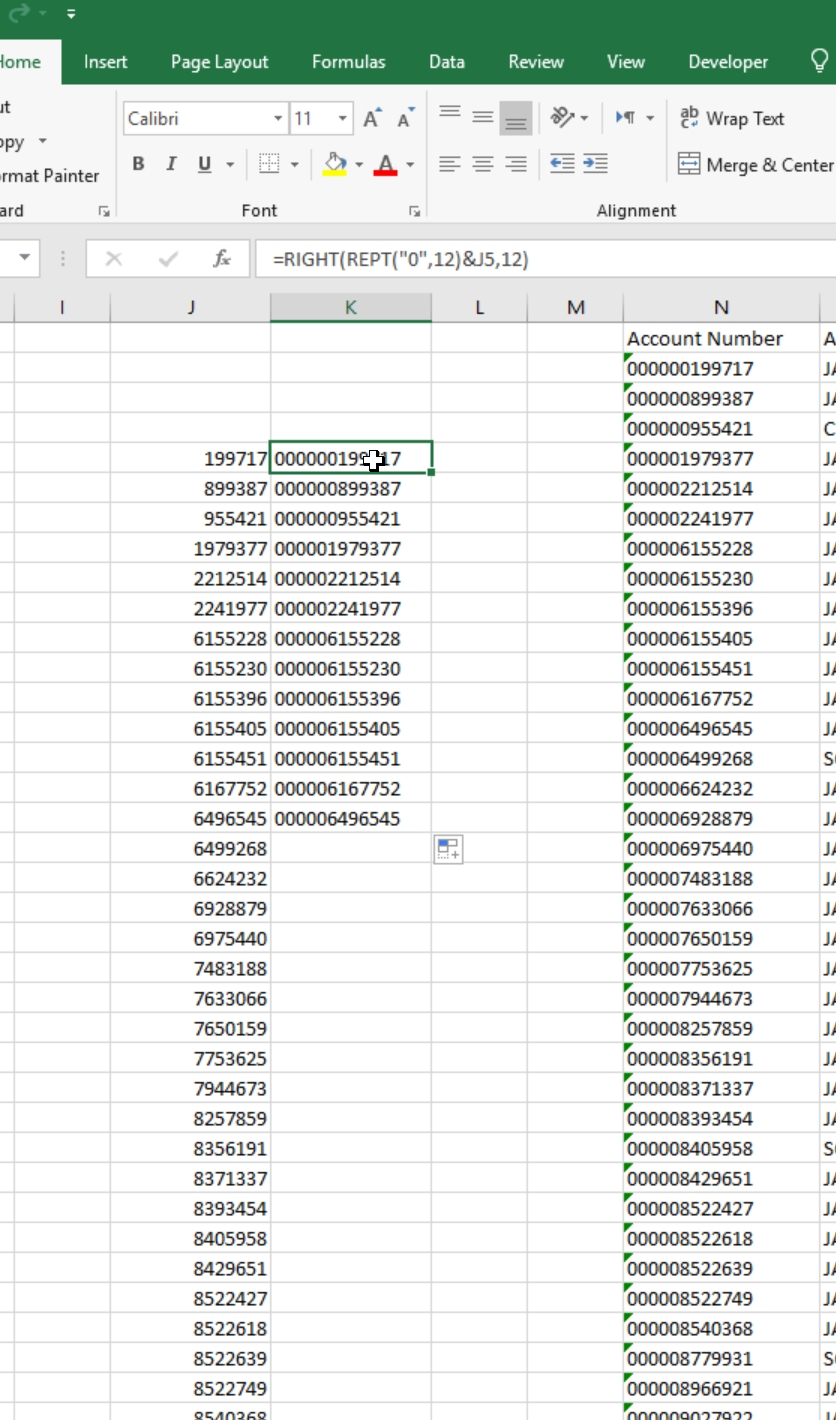# Fixed length IDI needed few months back to make a fixed-length ID iut if none-fixed-length numbers.

SO I used the function REPT with RIGHT to force all numbers to be converted into fixed-length ID (mainly having leading zeros)

And here is the formula

```=Right(Rept("0", 12)&J5, 12) ```

J5 is where the ID can be found

12 is the length of the ID we wanted